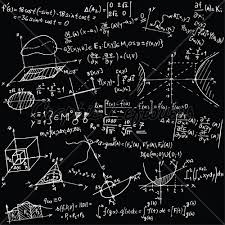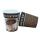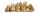# Equation 29

Solve next equation:

2 ( 2x + 3 ) = 8 ( 1 - x) -5 ( x -2 )

x =  0.7059

### Step-by-step explanation:

2 ( 2x + 3 ) = 8 ( 1 - x) -5 ( x -2 )

2•( 2•x + 3 ) = 8•( 1 - x) -5•( x -2 )

17x = 12

x = 12/17 ≈ 0.705882

Our simple equation calculator calculates it.Did you find an error or inaccuracy? Feel free to write us. Thank you!Tips to related online calculators
Do you have a linear equation or system of equations and looking for its solution? Or do you have a quadratic equation?

## Related math problems and questions:

• Simple equation 324 = n • 27, solve for n
• Find xSolve: if 2(x-1)=14, then x= (solve an equation with one unknown)
• Equation 25Solve following simple equation: 3/4(x+5)=1/2(x+9)
• Equation 11Solve equation: 0=y-1,2.(y-1,5)
• Simple equation 9Solve the following equation: -8y+5=-9y+9
• The math testThe math test contains 20 problems. For each correctly solved problem, the solver gets 3 points, for each incorrectly solved or unsolved problem, 2 points are deducted. Ondrej got 25 points. How many problems did he solve correctly?
• Equation 15Solve equation with variables on both sides:
• Negative in equation2x + 3 + 7x = – 24, what is the value of x?
• Simple equationSolve the following simple equation: 2. (4x + 3) = 2-5. (1-x)
• EquationSolve equation and check the result: 1.4x - 3/2 + x - 9,8 = x + 0,4/3 - 7 + 1,6/6
• Volume of cubeSolve the volume of a cube with width 26cm .
• RegroupingSubtract mixed number with regrouping: 11 17/20- 6 19/20
• Reciprocal equation 3Solve reciprocal equation: 1/2 + 2/3=1/x
• Unknown number 6Determine x if 1/6 of x is equal to 2/5 of the number 24.
• CoffeeCoffee from the machine in the cup cost 28 cents. Coffee is 20 cents more expensive than the cup. How much is the cup?
• TwentyTwenty rabbits are put in 4 cells so that there are a different number of rabbits in each cell contains at least 3 rabbits. What is the largest possible number of rabbits in one cell?
• Find the 24Find the difference between 2/7 and 1/21